# How do species coexist

### Competition of species and conditions for coexistence

Interaction between two populations

If two populations of different species have any influence on one another, then the effect of one species on the other can be positive (+) or negative (-); the neutral influence (0) is not taken into account here. If both types have a negative influence on each other (-), one speaks of Competitor, with mutually positive influence (++) of cooperation or symbiosis. If the relationship is positive for one population and negative for the other population (+ -), then we have one Predator-prey relationship or with Parasitism to do.

The following two examples of competitive relationships have been examined more closely in laboratory experiments (Odum, 1991, 179 ff.).

Flour beetle

: If you put two kinds of flour beetle in a container with flour, one kind will die out sooner or later. Two species cannot survive in the same container, even if each thrives well on its own under the given conditions. The relative number of individuals that are initially introduced into the mixed culture does not affect the end result. One species prevails in hot and humid conditions, whereas the other wins when it is cool and dry. We are dealing with a competitive relationship in which coexistence does not seem possible.

Clover varieties:

Two species of clover planted closely together in bowls are able to fully go through their life cycle and produce seeds, although the density of each species is lower than in pure cultures. Small but decisive differences in the forms of growth make this possible Coexistence of competitors. One species grows faster and is more likely to reach the maximum leaf density. In contrast, the other species has longer petioles and their leaves are higher up; so this species can prevent dying in the shadow of others.

We want to set up growth equations for two populations that are in competition with one another. A simulation is to show whether the behavior observed in rice flour beetles and clover varieties can be reproduced.

The Competition equations arise when looking into the law of growth for the stock N1 of population 1 is the population-dependent growth rate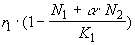begins. The law of growth for the stock is obtained accordingly N2 of population 2 if one considers the population-dependent growth rate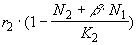chooses. The constants r1and r2 are the basic growth rates of the populations. The constants K and K are capacities of the environment for populations 1 and 2, each on the condition that they occupy the habitat alone. The parameter (the constant) is the Competition coefficient of type 2 with respect to type 1 and the parameters ß is the competition coefficient of type 1 with respect to type 2. The model is implemented in the Excel worksheet Competition.

#### balance

Now we can experiment with the model. We first want to find out to what extent the observed competitive behavior can be simulated with this model. Since we want to use the negative method, we basically start with a guess, which we then test by experiment.

Assumption 1:

The exclusion of competition observed in rice flour beetles is due to the fact that the basic growth rates r1 and r2 are different. We assume that otherwise each individual in its own population changes the growth conditions in the same way as an individual in the other population. This corresponds to the competition coefficients = ß = 1. The capacity is also the same for both populations.

Experiment:

We carry out the simulation with the parameters in the table below and find that the two types can coexist. There is no such thing as the presumed exclusion of competition, regardless of the population size you start with.

Analysis:

A decision between the two populations is not to be expected in the area of ​​r-growth: Both populations grow, one just a little faster than the other. The decision would have to come near the capacity limits. But: if one of the populations grows, the other grows too. The reduction of the growth rates close to the capacity limit reduces the stock-dependent growth rates of both populations equally. The observed behavior of the model now appears plausible. We reject the assumption.

Assumption 2:

One population displaces the other when it can make better use of the habitat, i.e. when the capacity of the environment is greater for it than for its competitors.

Experiment:

We choose the following parameters.

The simulation result can be seen in Figure 6. We find that the predicted exclusion of competitors actually occurs. Population 2 disappears even if its initial population is much larger than that of population 2 (as seen in the picture).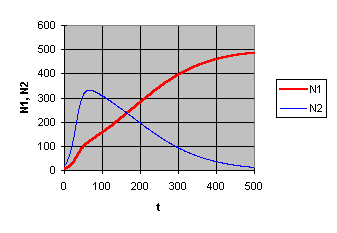Pic 6

Exclusion of competition through different capacities

Analysis:

The behavior observed in the model corresponds to that observed in real living beings in the laboratory. That confirms our assumption and also the chosen model.

Assumption 3:

The coexistence observed in the clover varieties is due to the fact that the interaction between the populations is comparatively low. This means that the competition coefficients are less than zero.

Experiment:

We choose the parameter values ​​from the following table. In the experiment, the assumption is confirmed: Independent of the initial populations, the same equilibrium value occurs again and again, which is 385 for population 1 and 231 for population.

Analysis:

The assumption is confirmed. But beyond that, the experiments made us curious. We are interested in where it comes from that the same final values ​​occur over and over again in our model. It must be a stable state of equilibrium. The so-called state diagram (here N-N-Diagram). It can be seen in Figure 7 (left half of the picture). (The usual t-N-Diagram is to the right.)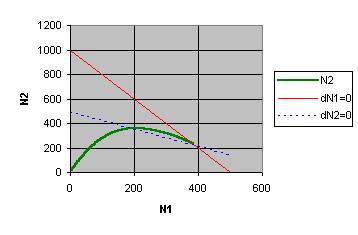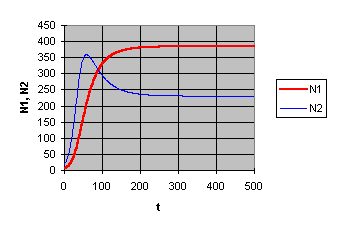Coexistence: a stable equilibrium

They are drawn in Zero growth curves dN1= 0 for population 1 and dN2= 0 for population 2. You are through the equations N + N = K (for dN1= 0) and N + ßN = K (for dN2= 0) given. These equations yield pairs of values ​​(N1, N2), for which the respective population stagnates, i.e. has a growth rate equal to zero.

At the intersection of the two curves there is equilibrium: both populations stagnate. The following formulas result for the equilibrium values ​​of the populations: N = (K - K)/(1-ß) and N = (K - ßK)/(1-ß).

#### coexistence

The negative method promotes our understanding of the theory. In the end it brought us to a general law that was new to us. We're trying to generalize the law. We make the following bold hypothesis.

Assumption 4:

Whenever the zero growth curves intersect in the positive area (i.e. for population sizes greater than zero), we are dealing with a stable equilibrium. There is coexistence in the equilibrium point.

Experiments:

We try out several parameter combinations and find that the assumption is often confirmed. But there are counterexamples. For example in the following case.

Only if both populations are started with exactly the same initial values ​​does the development approach the equilibrium point. If the initial values ​​deviate slightly from one another, this slight deviation remains in the area of ​​the r-growth.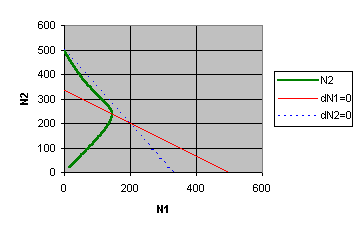Picture 8

Exclusion of competition: an unstable equilibrium

In the vicinity of the intersection of the zero growth curves, there is a radical change in behavior: the somewhat larger population emerges victorious from the competition within a short period of time. The other is completely suppressed. If population 2 has a slight start-up advantage, it will ultimately also prevail, Figure 8.

Analysis:

It is not only important that the zero growth lines intersect in the positive area. So the guess is not precise enough.

How can the presumption be made more precise? Perhaps we will get on with simple plausibility considerations. One consideration is that coexistence could arise if each population largely leaves the other alone, i.e. if the competition coefficients are relatively small. We make the assumption more precise: Coexistence is possible if and only if each additional individual in a population reduces its own habitat by a higher fraction than that of the competing population.

This can be expressed mathematically: The habitat (the growth rate) of population 1 is increased by a fraction of 1 /K reduced. Each member of the competing population 2 reduces the habitat of population 1 by /K. For the competitive population the corresponding values ​​are 1 /K or. ß/K. The conditions for coexistence can thus be specified as follows: /K < 1/Kand ß/K < 1/K. We come to the somewhat reformulated

Assumption 5:

The conditions for coexistence are K < K/and K < K/ß.

Experiments:

We do several experiments. All confirm the assumption made.

Analysis:

Since this is a general statement, one cannot be satisfied that it is confirmed by a few experiments. We are looking for a general justification. We draw the zero growth line in N-NChart a. The values ​​compared in the inequalities are exactly the axis intercepts of this straight line, Figure 9.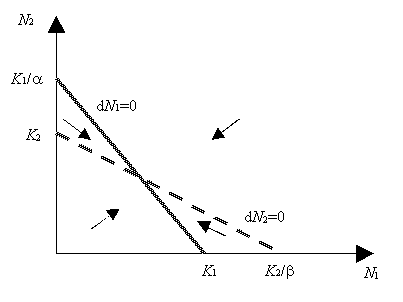Picture 9

Illustration of the condition for stable equilibrium

The qualitative system behavior can be easily determined using the zero growth curves: Below dN1 = 0 the population 1 grows, above it it shrinks. The same applies to population 2 with respect to the zero growth line dN2 = 0. If the zero growth lines intersect, there are four areas in the state space. These can be distinguished by the growth directions of the populations. Figure 9 shows the case that the conditions for coexistence set out above are met. It is easy to see that the population growth must basically lead to the intersection of the straight line.

#### Suggestions for discussion

1. The competition of species is one of the essential prerequisites for any evolution. Selection occurs when several species compete with one another for the same resources and when these resources are scarce in relation to demand. In short: selection is the result of competition for limited living space.

2.

Exclusion of competition and ecological niches: If two competing species are too similar in their needs, sooner or later one of them will be destroyed (exclusion through competition). If the ecological niches (i.e. the demands on nutrition and other environmental factors) of the two species are sufficiently different from one another, then they can coexist.

3.

What are the conditions for exclusion from competition? Conduct experiments to verify the assumption. Note: It is best to assume a logical negation of the conditions for coexistence.

4.

The simplifications of our model: restriction to a few aggregated sizes and effects ("reductionist view"), simplification of the functional relationships, deterministic approach. What additions and expansions in methodological terms and in relation to the object of consideration are available?

Back to the outline

© Timm Grams, July 6th, 1999